Origami-Instructions.com      spreading joy one fold at a time

Custom Search

## Like Origami? Tell your friends!

##### Most Popular Origami

These are currently our most popular origami:

#### Origami Paper

We use standard size 6 inch x 6 inch (15cm x 15cm) square origami paper for this site unless stated otherwise. If you can, use different types of origami paper to change the look of the finished origami and have fun with it!

# How to Divide Paper into Fifths

While you could divide a paper by measuring and then dividing it, there's also another way that's based on geometry. The math and origami masters have figured it out. We don't get into the gritty math details here. We just show you how to fold it!

Divide Square Paper into Fifths Step 1: Start with a 6 inch x 6 inch (15cm x 15cm) square paper.

Fold paper into half on the horizontal axis. Crease well and unfold.Divide Square Paper into Fifths Step 2: Make a diagonal fold from the corner to the center crease. Use a ruler to guide you if needed. Be as precise as possible. Crease well.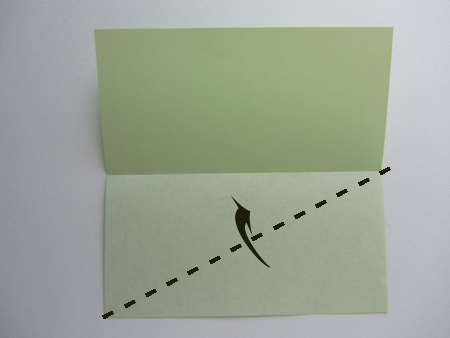Divide Square Paper into Fifths Step 3: We need to mark the spot where the tip touches. Make a slight horizontal and vertical crease on that spot. Once again, use a ruler if you need to.closer look...Divide Square Paper into Fifths Step 4: Unfold Step 2.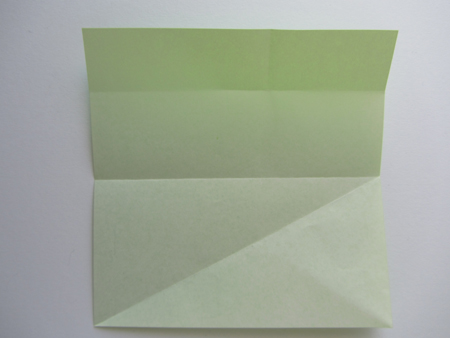Divide Square Paper into Fifths Step 5: Go ahead and extend those partial creases you made in Step 3. Fold, crease well and unfold.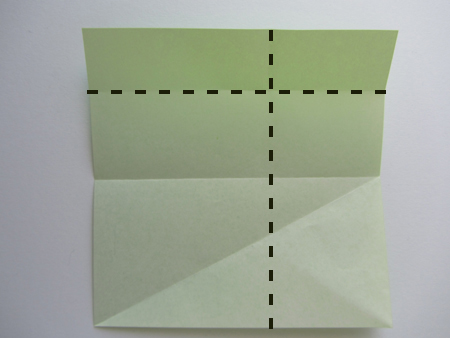Divide Square Paper into Fifths Step 6: What has happened is that by completing Steps 2 to 5, we've already divided the paper into fifths.

The math and origami masters have figured by making those folds, the top horizontal portion is now 1/5 of the paper.

And the right vertical portion is now 2/5 of the paper.

With this info, we can now proceed to divide the rest of the paper up into fifths.Divide Square Paper into Fifths Step 7: We'll divide the horizontal portions first. The photos may look confusing but it's actually pretty simple. Think of it this way....we need to divide the area A-B-C-D into 4 portions. We do this all the time right?

Fold A-B to C-D. Crease well and unfold.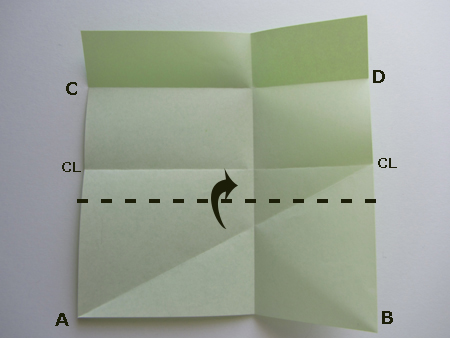Divide Square Paper into Fifths Step 8: Fold A-B to E-F.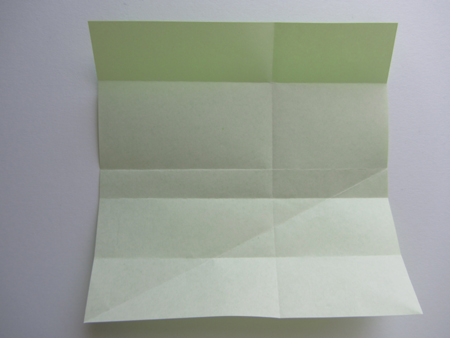Divide Square Paper into Fifths Step 9: Fold G-H to I-J. Crease well and unfold.We've completed dividing the paper into fifths on the horizontal side!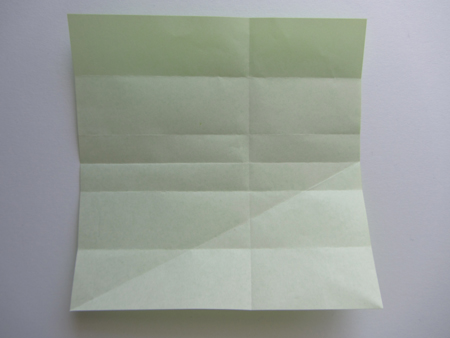Divide Square Paper into Fifths Step 10: Let's work on the vertical side.

Fold U-V to S-T. Crease well and unfold.As mentioned in Step 6, the area S-T-U-V is 2/5 of the paper.

So, U-V-W-X and S-T-U-V are now 1/5 each of the paper.Divide Square Paper into Fifths Step 11: The next steps are to divide the area
Q-R-X-W into 4 portions
. Think about it before you look at the photos below.

Fold Q-R to W-X. Crease well and unfold.Divide Square Paper into Fifths Step 12: Finally, fold Q-R to Y-Z. Crease well and unfold.and you've completed dividing your paper into fifths!